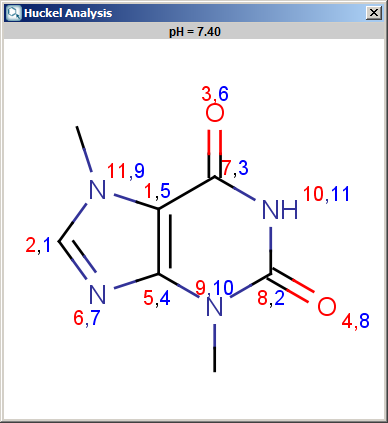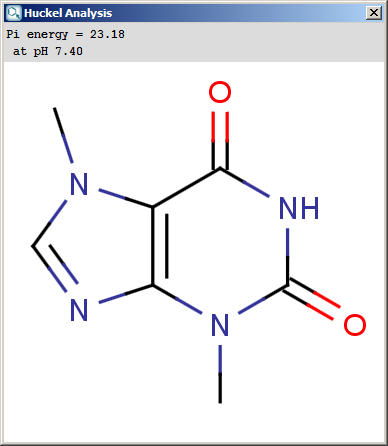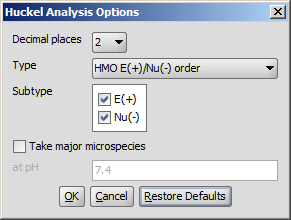Hückel Analysis Plugin

This manual gives you a walk-through on how to use the Hückel Analysis Plugin:

Introduction

The Hückel Analysis Plugin is able to calculate different structural descriptors for aromatic atoms.

Localization energies L(+) and L(-) for electrophilic and nucleophilic attack at an aromatic center are calculated by the Hückel method. A smaller L(+) or L(-) value means more reactive atomic location. Order of atoms in E(+) or in Nu(-) attack are adjusted according to their localization energies. The total π energy, the π electron density and the total electron density are also calculated by Hückel method. Depending on the chemical environment, the following atoms have optimal Coulomb and resonance integral parameters: B, C, N, O, S, F, Cl, Br, I.

All other atoms have a default, non-optimized parameter. Theoretical background is taken from Isaacs' book. Additional literature for the Hückel's parameters is Streitwieser's book.

The results appear in a new window, indicating the calculated values at the atoms in the aromatic ring. In the following example the first picture is the result of Aromatic E(+)/Nu(-) order calculation, while the second picture is the result of a Pi energy calculation.Fig. 1 Aromatic E(+)/Nu(-) calculation resultFig. 2 Pi energy calculation result

Options

The following calculation parameters can be set in the Huckel Analysis Options window:

• Decimal places: setting the number of decimal places with which the result value is given.

• Type: sets the type of the calculation. That could be:

• E(+)/Nu(-) order: numbers the aromatic atoms according to their probability of being attacked by electrophiles or nucleophiles.

• Localization energy L(+)/L(-): gives the localization energies of the aromatic center (dimension β).

• Pi energy: calculates the π energy of the aromatic ring(s) (dimension β).

• Electron density: calculates the π electron density.

• Charge density: calculates total charge density on the ring atoms.

• Subtype: E(+) or Nu(-): for E(+)/Nu(-) order and localization energy L(+)/L(-), the electrophilicity and nucleophilicity approaches can be selected (at least one of them). Results for E(+) are coloured red, and Nu(-) blue.

• Take major microspecies at pH: calculates the values for the major microspecies at the given pH.Fig. 3 Huckel Analysis Options window

References

1. Isaacs, N.S., Physical Organic Chemistry, John Wiley & Sons, Inc., New York, 1987, ISBN 0582218632.

2. Streitwieser, A., Molecular Orbital Theory for Organic Chemists, John Wiley, 1961, ISBN 0471833584.